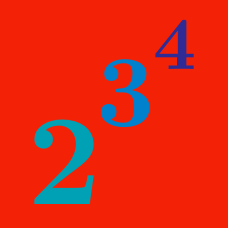Algebra

# Rules of Exponents: Level 1 Challenges

$\large \color{#D61F06}{2000}\left(\color{#3D99F6}{2000}^{\color{#20A900}{2000}}\right) = \, \color{#69047E}?$

If $-1 < x < 0,$ then what is the correct ordering of $x, x^2, x^3,$ and $x^4?$

True or false:

$\huge \displaystyle \sqrt{3^{3^3}} = 3^3 .$

Evaluate $\large 16^{0.125} - (0.5)^{-0.5}$

$\huge 2^{2^{2^2}} = \ ?$

×

Problem Loading...

Note Loading...

Set Loading...